# Python | Pandas Index.append()

Python is a great language for doing data analysis, primarily because of the fantastic ecosystem of data-centric python packages. Pandas is one of those packages and makes importing and analyzing data much easier.

Pandas` Index.append()` function is used to append a single or a collection of indices together. In case of collection of indices, all of them gets appended to the original index in the same order as they are passed to the `Index.append()` function. The function returns an appended index.

Syntax: Index.append(other)

Parameters :
other : Index or list/tuple of indices

Returns : appended : bool or array_like (if axis is specified)
A single element array_like may be converted to bool.

Example #1: Use `Index.append()` function to append a single index to the given index.

 `# importing pandas as pd ` `import` `pandas as pd ` ` `  `# Creating the first Index ` `df1 ``=` `pd.Index([``17``, ``69``, ``33``, ``5``, ``0``, ``74``, ``0``]) ` ` `  `# Creating the second Index ` `df2 ``=` `pd.Index([``11``, ``16``, ``54``, ``58``]) ` ` `  `# Print the first and second Index ` `print``(df1, ``"\n"``, df2) `

Output :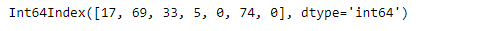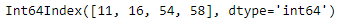Let’s append df2 index at the end of df1.

 `# append df2 at the end of df1 ` `df1.append(df2) `

Output :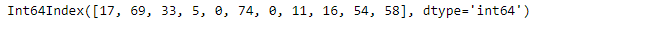As we can see in the output, the second index i.e. df2 has been appended at the end of df1.

Example #2: Use `Index.append()` function to append a collection of index at the end of the given index.

 `# importing pandas as pd ` `import` `pandas as pd ` ` `  `# Creating the first Index ` `df1 ``=` `pd.Index([``'Jan'``, ``'Feb'``, ``'Mar'``, ``'Apr'``]) ` ` `  `# Creating the second Index ` `df2 ``=` `pd.Index([``'May'``, ``'Jun'``, ``'Jul'``, ``'Aug'``]) ` ` `  `# Creating the third Index ` `df3 ``=` `pd.Index([``'Sep'``, ``'Oct'``, ``'Nov'``, ``'Dec'``]) ` ` `  `# Print the first, second and third Index ` `print``(df1, ``"\n"``, df2, ``"\n"``, df3) `

Output :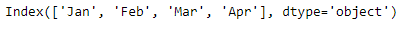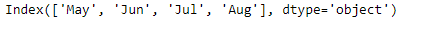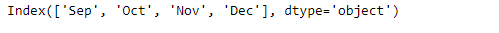Let’s append both the indexes df2 and df3 at the end of df1.

 `# We pass df2 and df3 as a list of ` `# indexes to the append function ` `df1.append([df2, df3]) `

Output :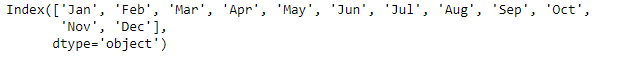My Personal Notes arrow_drop_upCheck out this Author's contributed articles.

If you like GeeksforGeeks and would like to contribute, you can also write an article using contribute.geeksforgeeks.org or mail your article to contribute@geeksforgeeks.org. See your article appearing on the GeeksforGeeks main page and help other Geeks.

Please Improve this article if you find anything incorrect by clicking on the "Improve Article" button below.

Article Tags :

Be the First to upvote.

Please write to us at contribute@geeksforgeeks.org to report any issue with the above content.